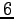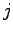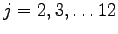1. Suppose a family has four children.
1. What is the sample space?
2. What is the probability of 4 boys? 4 girls?
3. What is the probability of 1 girl and three boys? 1 boy and three girls?
4. What is the probability of 2 boys and 2 girls?
5. What is the sum of your answers in parts b through d?
2. What is the probability of a family of three children consisting of two boys given that at least one of them is a boy? (Assume the probability of a boy child is the same as a girl child.)
3. A couple has two children. What is the probability that both are girls if the eldest is a girl? (Assume the probability of a boy child is the same as a girl child.)
4. What is the probability of flipping a coin four times and obtaining three heads, given at least two are heads?
5. A chairman and a vice-chairman of a board consisting of 10 men and 5 women are to be chosen. After a chairman is chosen, what is the probability that the vice-chairman is a woman?
6. Two fair dice are rolled. What is the conditional probability that the first one lands on 6 given that the dice land on different numbers?
7. What is the probability that at last one of a pair of fair dice lands ongiven that the sum of the dice is,.
8. Three cards are randomly selected, without replacement, from an ordinary deck of 52 playing cards. Compote the conditional probability hat the first card selected is a spade, given that the second and third cards are spades.

Math 804T
Experimentation, COnjecture, and Reasoning
Fall, 2007

This document was generated using the LaTeX2HTML translator Version 2002-2-1 (1.71)

Copyright © 1993, 1994, 1995, 1996, Nikos Drakos, Computer Based Learning Unit, University of Leeds.
Copyright © 1997, 1998, 1999, Ross Moore, Mathematics Department, Macquarie University, Sydney.

The command line arguments were:
latex2html conditional_probability_assignment

The translation was initiated by Steven Dunbar on 2007-11-30Steven R. Dunbar
Department of Mathematics and Statistics